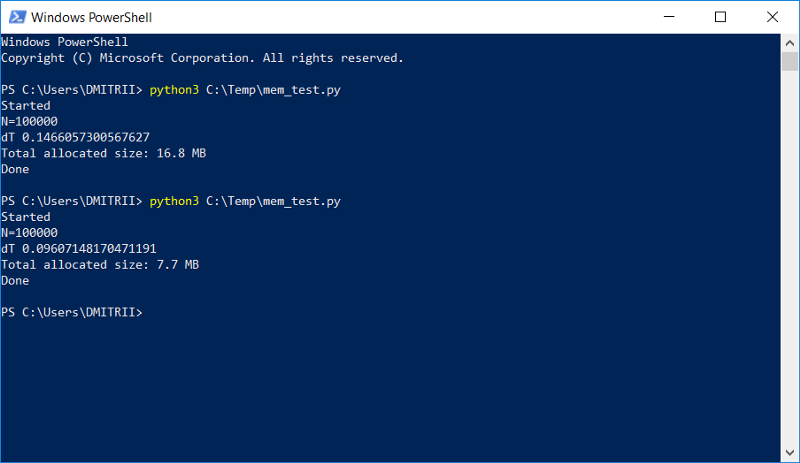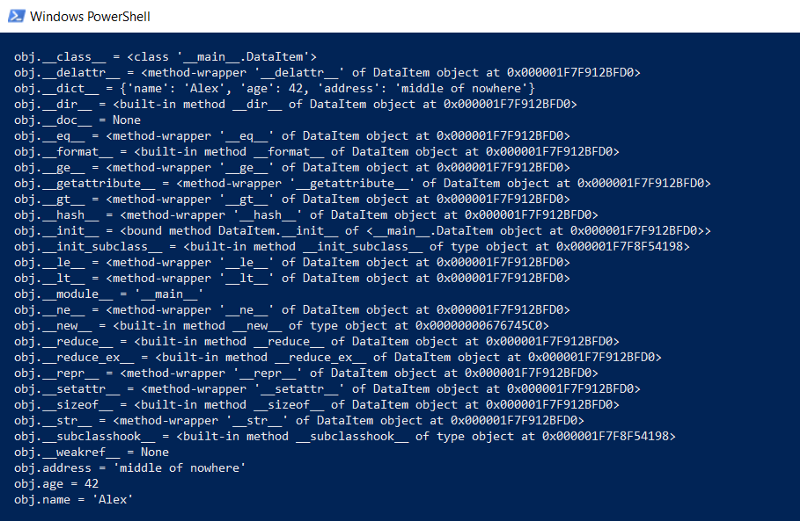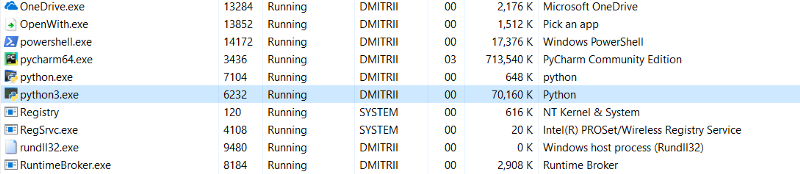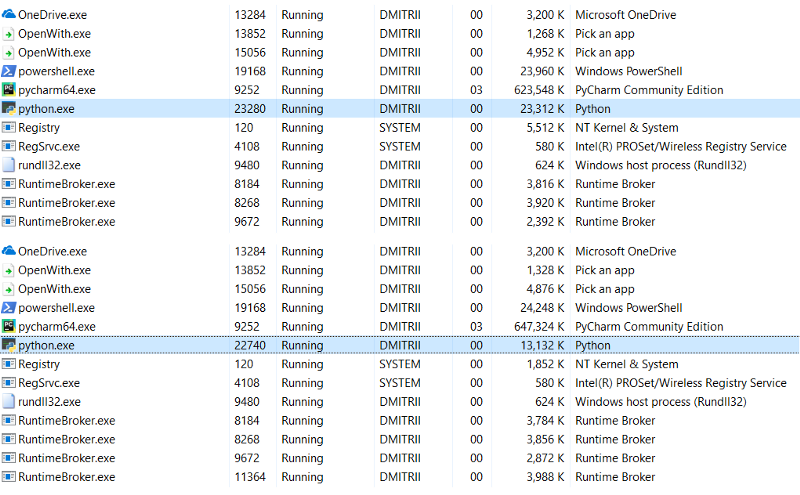# Python: How To Reduce Memory Consumption By Half By Adding Just One Line Of Code?Share :Originally posted on digitalminds.

I would like to share with you guys some sort of problem that we have experienced while me and my team work on one of the projects, where it was necessary to store and process a rather large dynamic list, testers began to complain about the lack of memory. And, the simple way to fix the problem with a little blood by adding just one line of code is described below.

### The result on the picture:How it works, I will explain down below. Consider a simple “learning” example — create a DataItem class that contains personal information about a person, for example, name, age, and address.

``````class DataItem(object):
self.name = name
self.age = age

### A question for beginners — how much does such an object take up in memory?

Let’s try the solution in the forehead:

``````d1 = DataItem("Alex", 42, "-")
print ("sys.getsizeof(d1):", sys.getsizeof(d1))``````

We get the answer 56 bytes. It seems a little, quite satisfied. However, we check on another object in which there is more data:

``````d2 = DataItem("Boris", 24, "In the middle of nowhere")
print ("sys.getsizeof(d2):", sys.getsizeof(d2))``````

The answer is again 56. At this moment we understand that something is not right here, and not everything is as simple as it seems at first glance.

Intuition does not fail us, and everything is really not so simple. Python is a very flexible language with dynamic typing, and for its work, it stores a lot of additional data. Which in themselves occupy a lot.

Just, for example, sys.getsizeof (“ ”) returns 33 — yes, as many as 33 bytes per empty line! And sys.getsizeof (1) will return 24–24 bytes for the whole number (I ask programmers in C to move away from the screen and not read further, so as not to lose faith in the beautiful). For more complex elements, such as a dictionary, sys.getsizeof (dict ()) returns 272 bytes — and this is for an empty dictionary. I will not continue any further, I hope the principle is clear, and the manufacturers of RAM need to sell their chips.

But back to our DataItem class and the “beginners” question.

### How much is this class in memory?

To begin with, we will output the entire contents of the class at a lower level:

``````def dump(obj):
for attr in dir(obj):
print("  obj.%s = %r" % (attr, getattr(obj, attr)))``````

This function will show what is hidden “under the hood” so that all Python functions (typing, inheritance and other buns) can function. The result is impressive:### How much does it all take up entirely?

On GitHub, there was a function that counts the actual amount of data, recursively calling getsizeof for all objects.

``````def get_size(obj, seen=None):
# From https://goshippo.com/blog/measure-real-size-any-python-object/
# Recursively finds size of objects
size = sys.getsizeof(obj)
if seen is None:
seen = set()
obj_id = id(obj)
if obj_id in seen:
return 0
# Important mark as seen *before* entering recursion to gracefully handle
# self-referential objects
if isinstance(obj, dict):
size += sum([get_size(v, seen) for v in obj.values()])
size += sum([get_size(k, seen) for k in obj.keys()])
elif hasattr(obj, '__dict__'):
size += get_size(obj.__dict__, seen)
elif hasattr(obj, '__iter__') and not isinstance(obj, (str, bytes, bytearray)):
size += sum([get_size(i, seen) for i in obj])
return size``````

Let’s try it:

``````d1 = DataItem("Alex", 42, "-")
print ("get_size(d1):", get_size(d1))

d2 = DataItem("Boris", 24, "In the middle of nowhere")
print ("get_size(d2):", get_size(d2))``````

We get 460 and 484 bytes, respectively, which is more like the truth.

With this function, you can conduct a series of experiments. For example, I wonder how much space the data will take if the DataItem structures are put in the list. The get_size ([d1]) function returns 532 bytes — apparently, these are the “same” 460 + some overhead. But get_size ([d1, d2]) returns 863 bytes — less than 460 + 484 separately. The result for get_size ([d1, d2, d1]) is even more interesting — we get 871 bytes, only slightly more, i.e. Python is smart enough not to allocate memory for the same object a second time.

Now we come to the second part of the question.

### Is it possible to reduce the memory consumption?

Yes, you can. Python is an interpreter, and we can expand our class at any time, for example, add a new field:

``````d1 = DataItem("Alex", 42, "-")
print ("get_size(d1):", get_size(d1))

d1.weight = 66
print ("get_size(d1):", get_size(d1))``````

This is great, but if we do not need this functionality, we can force the interpreter to specify the list of class objects using the __slots__ directive:

``````class DataItem(object):
self.name = name
self.age = age

More information can be found in the documentation (RTFM), in which it is written that __ dict__ and __weakref__. The space saved over using dict can be significant. We check: yes, indeed significant, get_size (d1) returns … 64 bytes instead of 460, i.e. 7 times less. As a bonus, objects are created about 20% faster (see the first screenshot of the article). Alas, with real use of such a large gain in memory will not be due to other overhead costs. Create an array of 100,000 by simply adding elements, and see memory consumption:

``````data = []
for p in range(100000):
data.append(DataItem("Alex", 42, "middle of nowhere"))
snapshot = tracemalloc.take_snapshot()
top_stats = snapshot.statistics('lineno')
total = sum(stat.size for stat in top_stats)
print("Total allocated size: %.1f MB" % (total / (1024*1024)))``````

We have 16.8 MB without __slots__ and 6.9 MB with it. Not 7 times of course, but it’s not bad at all, considering that the code change was minimal. Now about the shortcomings. Activating __slots__ prohibits the creation of all elements, including __dict__, which means, for example, this code for translating structure into json will not work:

``````def toJSON(self):
return json.dumps(self.__dict__)``````

But it is easy to fix, it is enough to generate your dict programmatically, going through all the elements in the loop:

``````def toJSON(self):
data = dict()
for var in self.__slots__:
data[var] = getattr(self, var)
return json.dumps(data)``````

It would also be impossible to dynamically add new variables to the class, but in my case, this was not required. And the last test for today. It is interesting to see how much memory the entire program takes. Add an infinite loop to the end of the program so that it does not close, and look at the memory consumption in the Windows Task Manager.

### Without __slots__:6.9MB turned into 27MB … well, after all, we saved the memory, 27MB instead of 70 is not so bad for the result of adding one line of code. Notes: A lot of additional memory is used by the tracemalloc debug library. Apparently, she adds additional elements to each created object. If you turn it off, the total memory consumption will be much less, the screenshot shows 2 options:### What if you need to save even more memory?

This is possible using the numpy library, which allows you to create structures in the C-style, but in my case, it would require a deeper refinement of the code, and the first method would be quite enough. It is strange that the use of __slots__ has never been analyzed in detail on Habré, I hope this article will fill this gap a bit.

## Conclusion

It may seem that this article is an anti-Python ad, but it’s not at all. Python is very reliable (in order to “drop” a program in Python, you have to try very hard), an easy-to-read and convenient language for writing code. These advantages in many cases outweigh the disadvantages, but if you need maximum performance and efficiency, you can use libraries like numpy, written in C++, which work with data quite quickly and efficiently. Thank you all for your attention, and have a nice coding.

Source: digitalminds

Share :